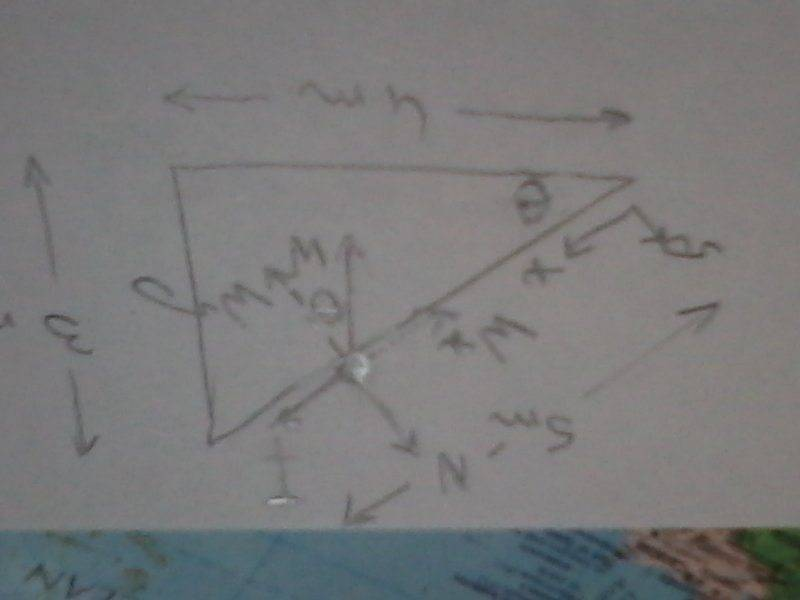# British Physics Olympiad 2004 (GCSE)

• LostAce

#### LostAce

5. Point Q is 3m above and 4m to the North of Point P. Ignoring friction how much energy does it take to move a 5kg sack of potatoes from point P to point Q?

These are multiple choice questions of the exam, so in the mark scheme it only says: A, B ...

I am looking for an explanation as to why I am wrong

My current working:
We can use the Pythogorean Theorem , to determine that the box has to be moved a diagonal distance of 5m. (Right/ Wrong and why?)
5kg can give an approximate value of about 50N (e.g. gravitaional field about 10 ms^2)(Right/ Wrong and why?)
Finally we can use the equation W = fxd = 50N x 5m = 250J(Right/ Wrong and why?)

But in the marscheme it says the solution is C, namely 150J...
Why?

Last edited:

The work done is used to raise the sack's potential energy.

This would mean I should calculate GPE only.? (e.g. 5 x 10 x 3 =150)
What about the other 4m? Further explanation please as to why I can neglect the other distance ( Diagram would be nice :D)

No forces acting horizontally on moving the sack.

Work and energy go hand in hand, but work do not have the normal intuitive meaning in physics.
Just carrying a heavy object over a flat surface requires no work.
In physics the work takes the direction of the required force to the direction in which the object is moved
into account like this: W = Fs cos(θ)
where W is the amount of work done by the force F to transport the object over a straight distance s and
θ is the angle between the direction of the force and the distance in which it is moved. In this case the force
needs to balance the object's weight out, so it is upwards, s is 5 meters and the cosine of theta is 3/5. So
W = mg5x3/5
This work is used to raise the sack's potential energy.

No forces acting horizontally on moving the sack.
Is this because friction is neglected? But If I push the sack of potatoes, surely there is a force acting on it?

Is this because friction is neglected? But If I push the sack of potatoes, surely there is a force acting on it?
You are thinking of the case of pushing the sack across a horizontal frictionless surface, right? Yes, you have to do work to accelerate the sack from rest up to some speed, but in this question there is no particular speed that has to be attained. The sack can be moved as slowly as you like, so the energy invested as KE can be as small as you like. What remains (in the OP) is the energy needed to raise the sack against gravity.
Re the reason your force times distance equation didn't work, see andrevdh's explanation, that the distance is the distance the force moves in the direction of the force.

Ignoring friction is a very difficult situation for us to envisage. How would you manage to push something up a sippery slope? Well it would not be possible. You would slide down. So this is a theoretical situation. Maybe we should think that it is pulled up with a rope, but since there is no friction countering its motion uphill, all the rope needs to do is raise it upwards, that is countering its weight.

Lets look at the forces on the sack on the inclineThere are 3 forces N, W and T, the tension in the rope pulling the sack uphill. I have chosen the x-axis along the incline and have resolved the weight into its 2 components.